## Scala: Tail Recursion Optimization and Comparison to Java

Tail Recursion is supposed to be a better method than normal recursion methods, but does that help in the actual execution of the method? We can only say yes if the recursion actually does not increase the call stack in memory and instead re-uses it.

It depends completely on the compiler i.e. whether the compiler is really optimizing the byte code for tail recursion functions or not. Let us consider our plain old factorial program using Scala.

The following Scala code calculates the factorial in the tail recursion process: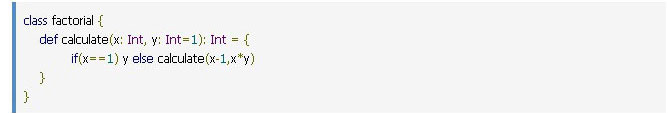Let us examine the byte code generated by the Scala compiler for the above Scala class. We write the above Scala code in a file, say “factorial.scala” and compile it using the command: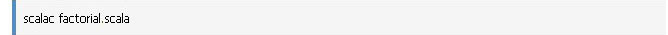This will generate the factorial.class file. We will now use the following command to inspect the byte code of the class file generated above: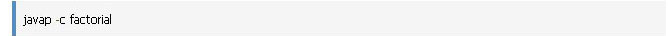This will give the byte code of the factorial.class which will look something like the below: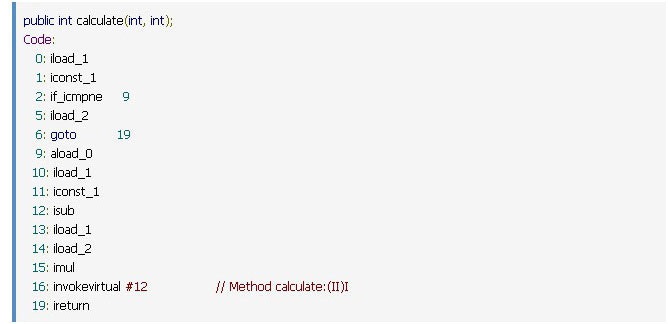(For details of the above JVM instruction set please refer to the Online Instruction Reference)

If we closely look into the byte code above, we will see that the calculate method is again calling itself – the invokevirtual #12 is actually calling the calculate method repeatedly for each recursion. This signifies that for each recursive call the calculate method is getting called which is in turn increasing the call stack in the memory.

So ideally we are not getting any advantage of tail recursion optimization even though Scala claims that it optimizes tail recursive function.

Why?

Let us re-write the factorial code as below: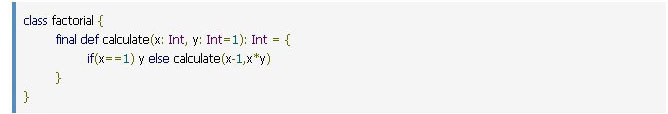Here we have added the final keyword before the method definition of the calculate method. We all know that adding the final keyword prevents this method from being overridden in the subclasses.

Now if we compile the above class and see the byte code, it will look something like this: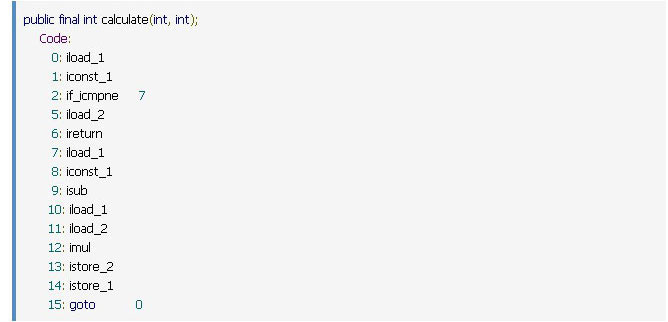We can see that the above byte code never calls the calculate method, instead, it calls the same instructions in a loop. This signifies that for the recursive call, the same method calculated is not getting called repeatedly, thus not increasing the call stack in the memory.

We can say now that the Scala compiler has optimized the tail recursive function.

But what is the reason for this difference? Scala compiler will optimize any tail recursion function only if it is sure that the same function will not be overridden. After all, any sub-class that overrides the function can change the implementation to a non-tail recursive code. Here we have achieved this by adding the final keyword. The other possible way is to make the function private, which will also prevent the function from being overridden; but this will also reduce the scope of that function. So, the decision to make it final or private will depend on the design of our code.

To ensure that the compiler optimizes the tail recursive function, we can add @tailrec annotation to the function that we want the compiler to optimize. The code will look something like below: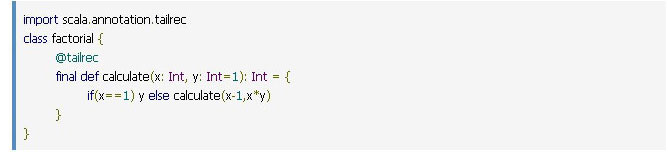In the above code if we remove the final keyword and try to compile the code using Scala we will get the following compilation error: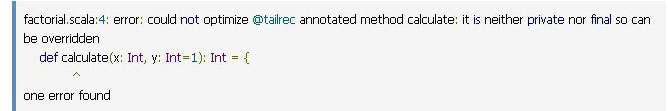This clearly indicates the reason why the Scala compiler did not optimize the calculate method in the first code snippet. If we remove the @tailrec annotation, the Scala compiler will generate non-optimized byte code which it did in our initial code at the beginning.

Now what about Java? We can write the same factorial code in Java using the tail recursive function as follows: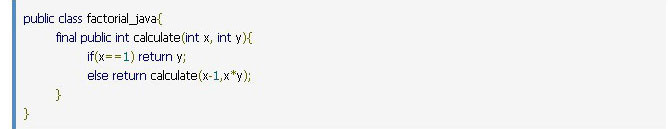Here we have added the final keyword again to ensure that it cannot be overridden in the sub-classes. Let us compile the above Java class: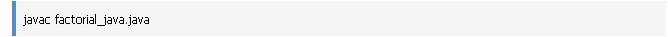If we investigate the byte code generated for the above Java class using the javap command we will get something like this: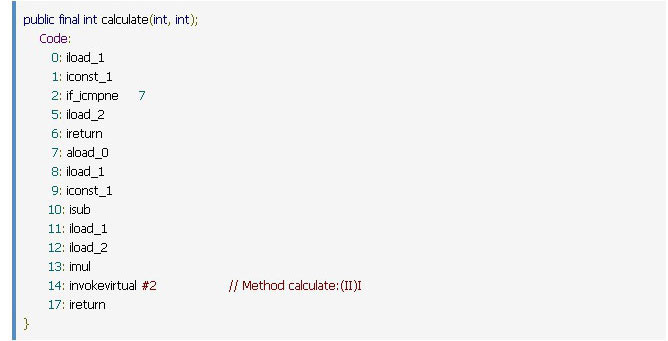Here we can see that the generated byte code calls the calculate method for each recursion which is similar to the one generated by the Scala compiler in our first example. So the generated byte code is not optimized for the tail recursive method and in turn, increases the call stack in memory. Even if we remove the final keyword from the calculate method in the Java class and generate the byte code we will see the same result.

This signifies that whatever may be the method declaration, the Java compiler will not optimize a tail recursive method. Whereas the Scala compiler will optimize the same if the method is declared as final or private.

We can thus say that a Tail Recursive function has no effect on performance in Java, whereas the Scala compiler will optimize tail recursive functions based on the condition that the code ensures that the function is not overridden in subclasses.

Author: Dipta P. Banerjee